# OECD Gross pension replacement rates data analysis 4 - Visualization Histogram and Boxplot using R. There is not much difference between 2014 and 2020.Generated by Bing Image Creator
www.crosshyou.info

This post is following of the above post. In this post I will analysis difference between 2014 and 2020.

First, I make a data frame to calculate difference between 2014 and 2020.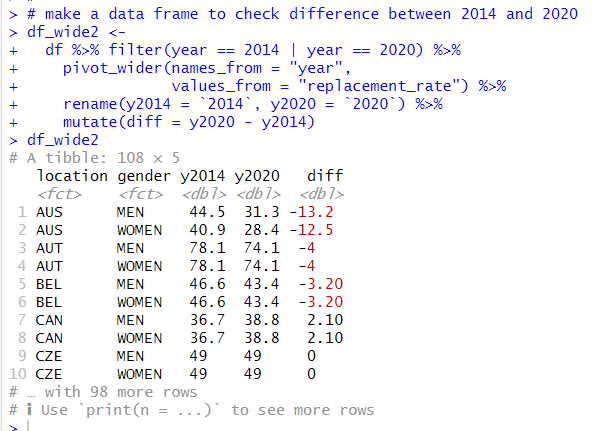filter() function makes observations for 2014 and 2020 only.

pivot_wider() function transform a data frame from long format to wide formant.

rename() function makes a new variable name.

Then, mutate() function makes a new variable, "diff", which is the replacement rates between 2014 and 2020.

I see some locations have negative value, somes have positive value.

Let's see summary statistics with summary() function.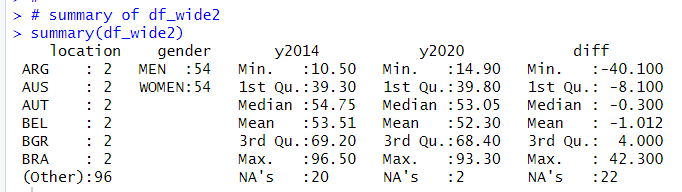Oh, there are some NAs. I will delete NA rows with na.omit() function.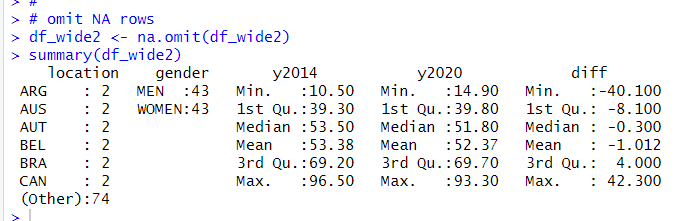I see mean diff is -1.012. Let's see a histogram of "diff".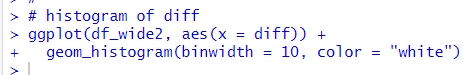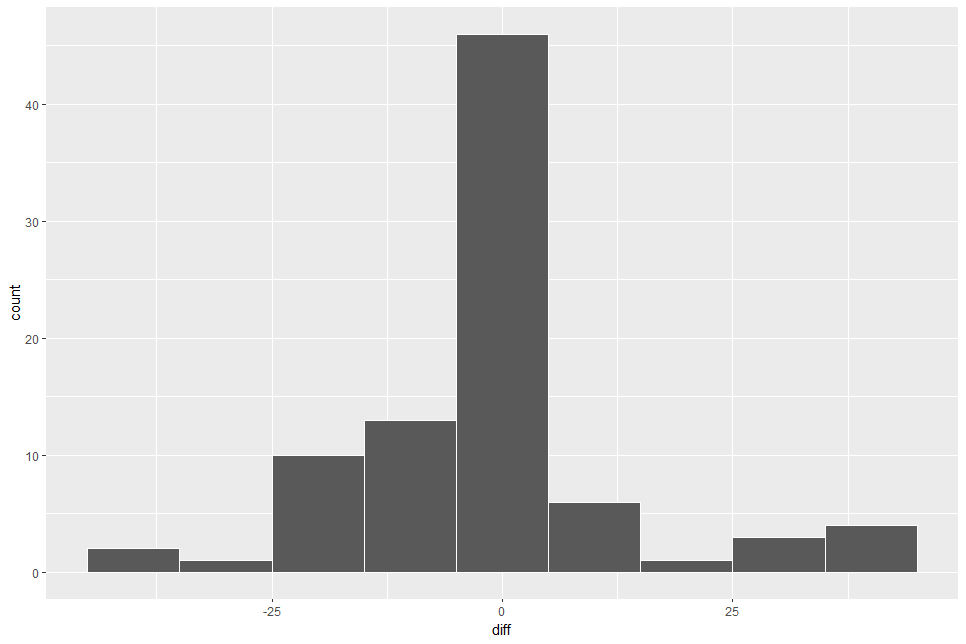Almost observations are at around 0.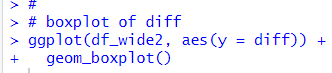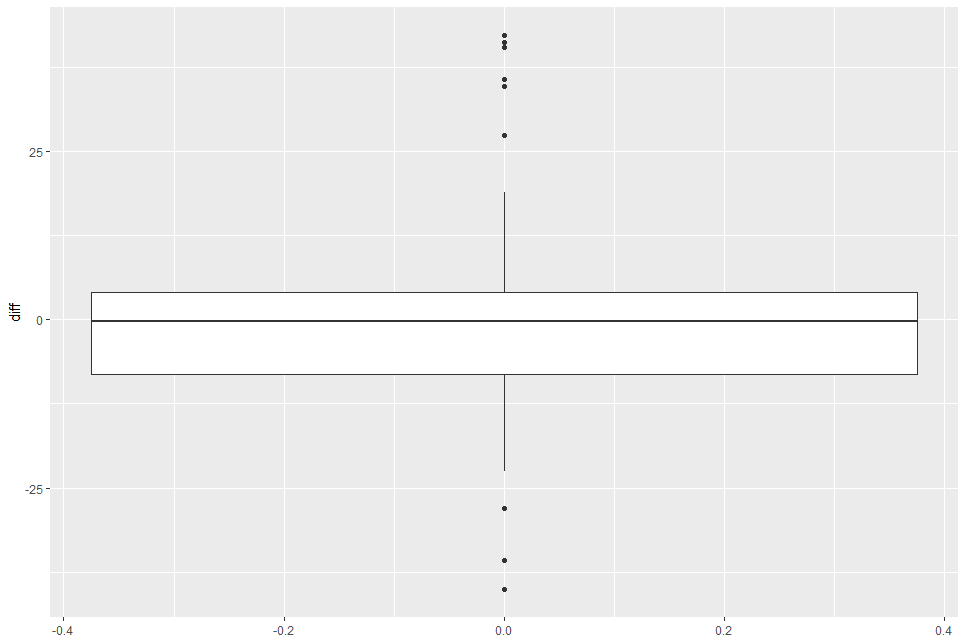Boxplot shows median value is near 0.

Let's see there is gender difference.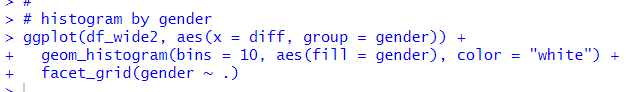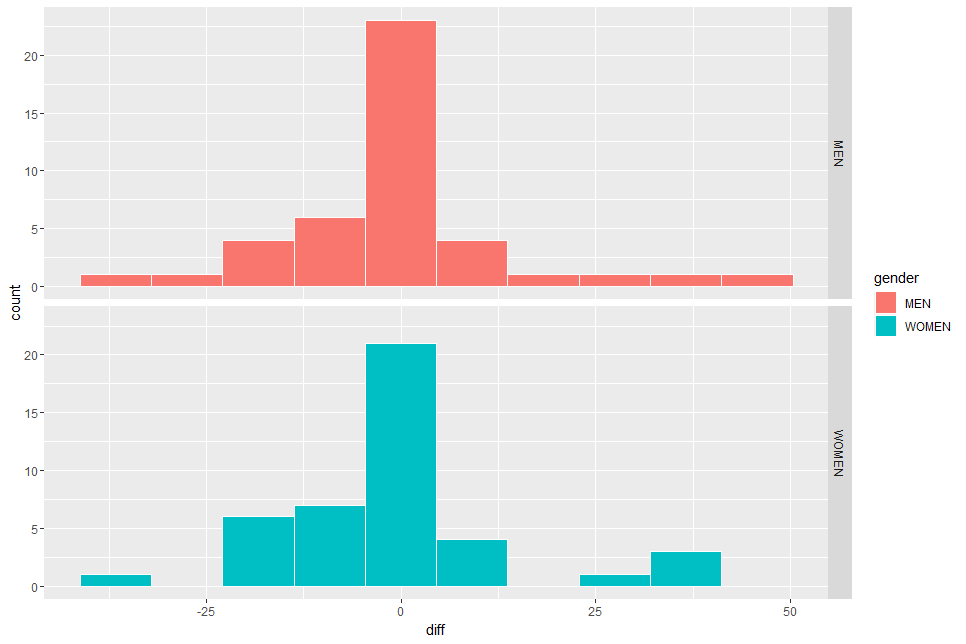I don't see there is much difference.

Let's use boxplot too.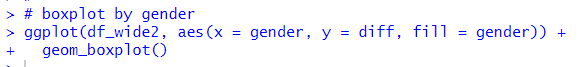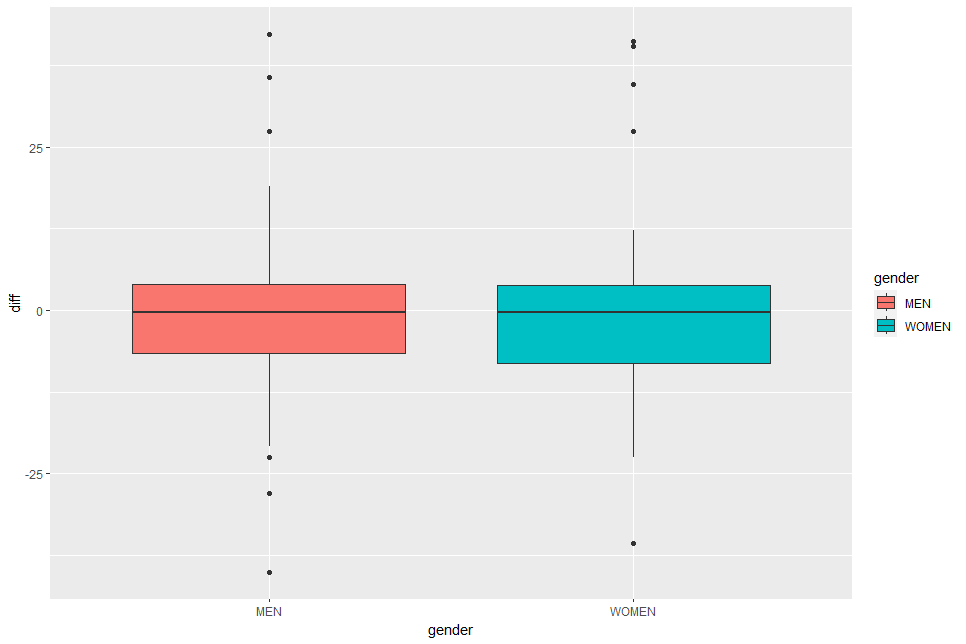In the above boxplot, I don't see much difference neither.

So, from the point of visualization view, I don't see the difference between 2014 and 2020, by gender, by overall.

That's it. Thank you!

Next post is,

To read from the first post,